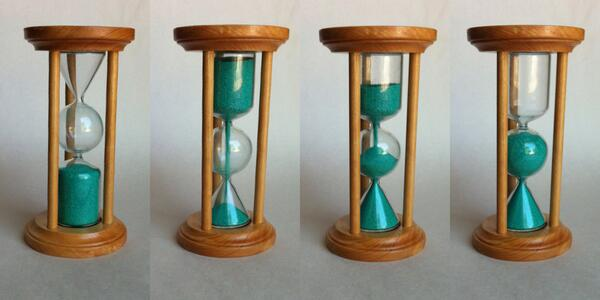09 Apr

So it became time to decide on Studio Infinity’s giveaway at the 13th Gathering for Gardner (G4G13). By tradition, at least, it’s considered a plus for giveaways to connect with the number of the conference — 13 in this case. So this mathematical free association starts with the number 13.

What thoughts does 13 evoke? First and foremost, it’s a Fibonacci number, 13 = 8 + 5. So something about breaking something down by addition? Addition is so simple, what could an interesting giveaway to do with addition be like? What if it’s not just addition of numbers, but addition of something more involved? Like addition of volumes? Is there an interesting instance of volumes adding up nicely?

Well, in fact there is: the so-called Archimedes hourglass, which I had seen people make and show at previous G4Gs, such as this beautiful example by Rod Bogart:The reason this works is that Archimedes determined that the volume of a cylinder of unit radius and two units height is equal to the sum of the volumes of a sphere of unit radius and a cone of unit base radius and two units height. Is there some variation on that which could be interesting? To try to find something like that, maybe we should look at how the proof of that fact goes.

But first, those occurrences of “two” seem a bit out of place. It seems a much more natural statement to say that the volume of a cylinder of unit radius and unit height is equal to the sum of of the volumes of a hemisphere of unit radius and a cone of unit base radius and unit height. And indeed, that statement has a simple and natural justification. Archimedes looked at it differently, but the 5th century Chinese mathematician Zu Gengzhi elucidated a principle (not rediscovered in Western mathematics until Bonaventura Cavalieri in the 17th century, and so sometimes called the “Cavalieri Principle”) that makes this straightforward, the Gengzhi Principle:

If the cross-sectional areas of two solids along every plane parallel to a fixed plane are equal, the solids have equal volume.

How does this apply to the cylinder, cone, and hemisphere? Let’s place them side-by-side this way:The yellow plane is at height h above the xy plane (light blue), which is the common base of all three volumes (well, actually, we’ve placed the apex of the cone at z=0). The yellow plane cuts the cylinder in a circle with radius 1, the cone in a circle with radius r, and the hemisphere in a circle with radius s. Moreover, since the width of the cone increases linearly from 0 at the blue plane to 1 at a height of 1, r = h. And since the equation of the red semicircle (which is the vertical cross-section of the hemisphere) is h² + s² = 1, we have that s = √(1-h²).

Therefore, the area of the horizontal cross section (in the yellow plane) of the cylinder is π·1² = π, while the areas of the cross sections of the other two solids are πh² for the cone and π(√(1-h²))² = π(1-h²). The latter two obviously sum to the former, since h² + (1-h²) = 1. Since this holds for any plane parallel to the light blue plane, we conclude by the Gengzhi Principle that the volume of the cylinder is the sum of the other two, just as Archimedes established. (Living six centuries earlier than and half a world away from Gengzhi, Archimedes of course had to rely on other methods, considerably more complicated, to justify this relationship.)

But now, as we will see in the next post, there is a great deal of flexibility in this proof of the Archimedes relationship — plenty to create an interesting G4G13 giveaway.